## Pages

### How to make a simple School Report Card in Microsoft Excel

In today's post, we are going to explore on how do we make a simple Excel Report Card for a given student with an existing marks dataset. We will also see how to apply our knowledge of formulas to calculate SUM, AVERAGE and also make a simple Bar Chart out of the data with the help of Excel recommendations. So let's get started.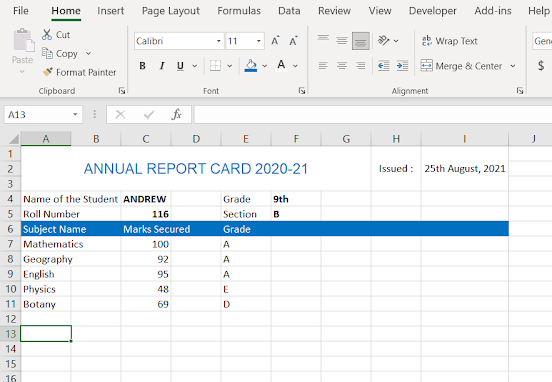A Basic Dataset Created in Excel for Students Marks and Grades

Now, you must be wondering, that what if there were too many marks in the dataset and we don't want to calculate the grades manually ? Well, Excel's got you a great solution to this problem.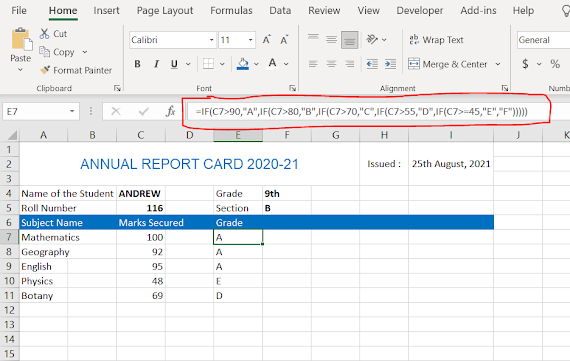An Example of NESTED IF Statement for using multiple criteria to find Grades

So, as you can see in the illustration above, we have got a formula in the formula bar. Let me explain. So, In the First IF, we have specified the criteria, C7>90, A, in the next IF, C7>80, B, and so on, until, we have exception that if the marks are below 45 the student gets an "F" grade.

Now, what if we want to calculate the SUM of the marks secured by Andrew in different subjects. It's simple, in the earlier post we have already learnt about SUM Function. So here we will use the same. Let's have a look in the next illustration.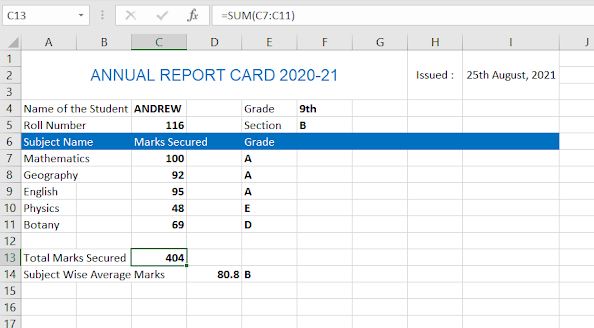Simple Application of SUM Function to Calculate the SUM of Marks from C7 to C11

So, in the above illustration, note that, in the formula bar, we have used the formula for calculating Total Marks Secured by the student in the data range C7 to C11 using the SUM(C7:C11) formula.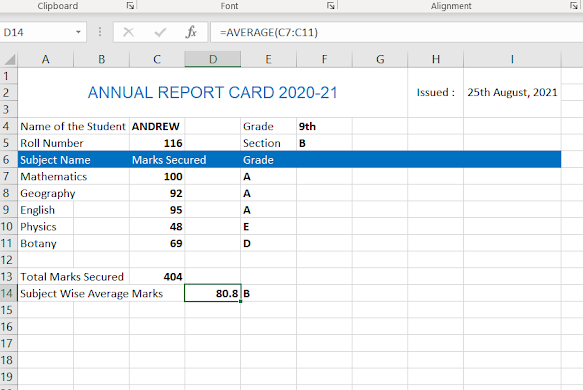Application of Formula for Subject Wise Average Marks of the Student

So, now we move ahead with the calculation of Average Marks of the student with the use of simple formula AVERAGE(Range of Data). So here in this case, in the formula bar we have entered AVERAGE(C7:C11). This easily gives us the average marks (subject wise) for the student Andrew. There is an alternate way to calculate the AVERAGE Marks of the Student with the traditional SUM/No. of Datasets formula. However, this formula is limited and does not automatically change with the change in dataset. Therefore, the EXCEL way of doing this using AVERAGE function is more secure way.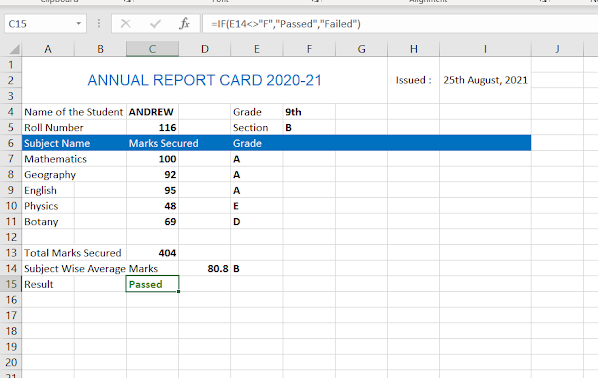Displaying Passed or Failed in Excel School Report Card

Further, if we want to declare whether the student is passed or failed, then we can simply use the IF statement and use the average marks or grade to declare. Here, I have used grade, so simple, if the grade is F, means the student is failed otherwise passed. So, please see the formula bar in the above screenshot to understand well.

Now, in order to calculate grade for Average marks only the range changes to D14 and all the earlier grade formula remains the same, as shown in the illustration below.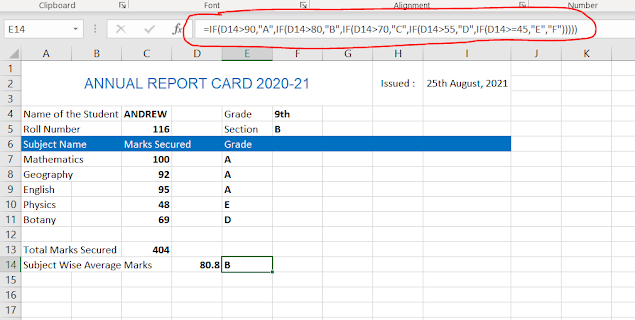Formula Change for Average Grade of the Student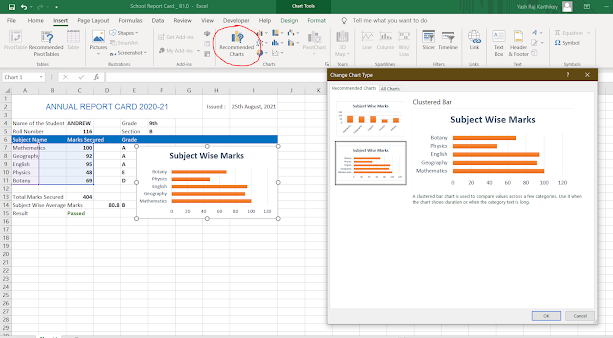Creating a simple chart of a given dataset using Recommended Charts Option in Microsoft Excel

So, in the above illustration as you can see, we selection the A7 to C11 as the data range for the chart. Now, we went to Insert tab and used Recommended Charts option as shown in the above figure, to create simple bar chart of the student performance. Here, we have two styles to show student marks, I chose the horizontal one.

So, in this way we have learnt to work hands on with Excel, and I expect that you will become more fluent on excel with help of this example. To sum up, we used Average, Sum, Nested If and Recommended Chart, formulas and option to create a simple report of the student Andrew, where the marks data was already given.

1.